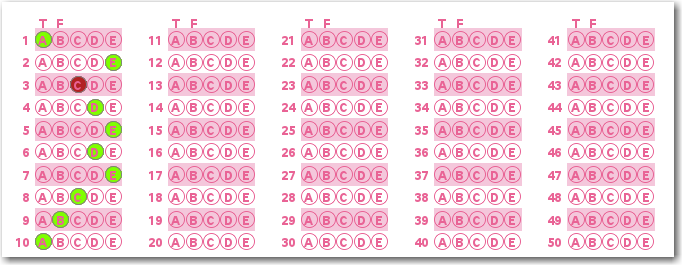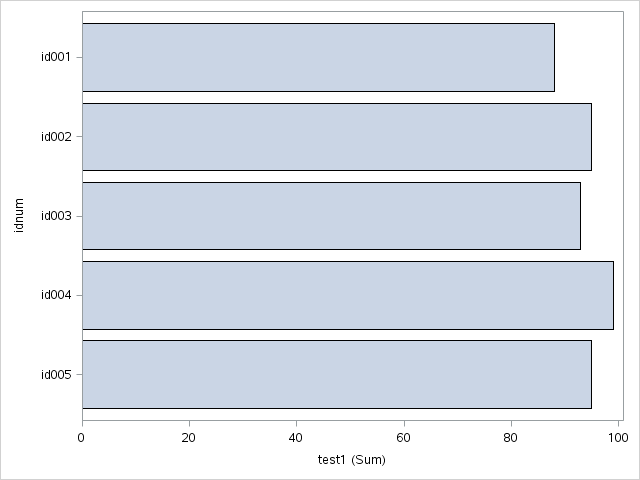Probably the most important thing you can learn in the free SAS University Edition is how to work with data. And one of the most powerful tools for working with data is Proc SQL ...

I've used Proc SQL in some of my previous blog posts for simple tasks (such as subsetting data), but this time we'll go a bit deeper and use it for something a bit more powerful - joining tables.

It is often the case that we maintain a data table with all the information about people (students, employees, customers, etc), and then in our daily transaction data we simply refer to them by some id number. That way we only have to maintain one copy of the people-data (name, address, age, gender, etc), we don't have to enter the same data multiple times (just the id number), and we don't have to store all that information for each transaction (only the id number).

In this example, I'm keeping the data very short and simple. We'll have a school class with 5 students, and the only data for each student is their name. Copy-n-paste the code into SAS University Edition and run it:

```data students; input idnum \$ 1-5 name \$ 7-50; datalines; id001 John Doe id002 Jane Doe id003 Raj Patel id004 Tran Park id005 Jet Lee ; run;```

Do you remember taking tests on Opscan sheets, with #2 pencils? For no particular reason, here's a visualization of one I created with SAS/Graph. This has nothing to do with the example, and is just here to jazz-things-up with a bit of color :)Now, let's assume we have a table of grades. For this very simple example, we'll say the students have only had one test so far. Notice in this table we only store the student id number (not the full name).

```data grades; input idnum \$ 1-5 test1; datalines; id001 88 id002 95 id003 93 id004 99 id005 95 ; run;```

If we want to see a bar chart of the grades, we can use the following simple code ... but it is difficult to tell which student is which, with only the student id numbers labeling each bar:

```proc sgplot data=grades; hbar idnum / response=test1; run;```And this is where the SQL join comes into play... You can use the following code to add the student name to the grades table. And while we're at it, let's order the data by the test1 score, so we can have the bars in ascending order:

```proc sql; create table plotdata as select unique grades.*, students.name from grades left join students on grades.idnum=students.idnum order by test1; quit; run;```

Now when we plot the data, we can label each bar with the student name, and order the bars by the data-order:

```proc sgplot data=plotdata; hbar name / response=test1; yaxis discreteorder=data; run;```Remember - this is a simplified example, just to demonstrate the technique of SQL joins. Now, use your imagination and come up with ways to apply this technique to other data you might have, and you will soon become a highly paid SQL expert! :)

Share